# On feeling stupid, how mathematics is taught in school, and an Ikea bowl

This story begins with a tweet: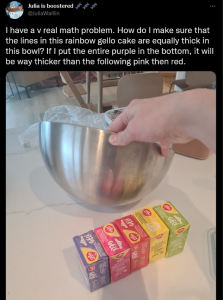In other words: how to find the quantity (volume) of each layer of jelly to make sure each layer in a bowl is of equal height?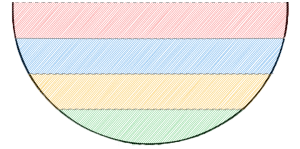Initially, I thought of a quick solution: mark height levels every n cm on the bowl with a pen, and then just fill up to each mark with the different liquid. There won’t be any need to calculate anything.

This can work assuming the bowl is made of glass. Maybe one could mark the bowl on the outside and then fill it up and still have a sufficiently clear reference for when to stop.

But… this didn’t feel satisfying. How would one approach the problem if they had to solve it without filling the bowl, with mathematics only?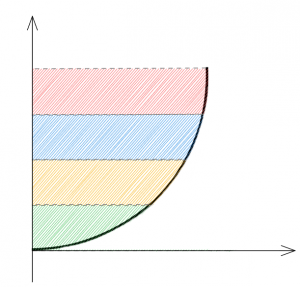One approach could be to find the area – labeled as B below – between the X axis and the curve of the “bowl”.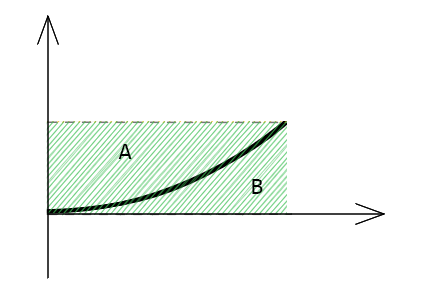We know what A + B is, that is, the height of the layer we desire, multiplied by the width of the bowl at that point. From that area (the green colored above) we subtract B, and we find A, the area of the liquid.

Can we then find the curve or mathematical function that characterizes our particular bowl? How do we find it? I tried to use different methods, unsuccessfully, for instance tracing the bowl profile on a sheet of paper. In the end, I used my phone to take a picture of the bowl, a common Ikea metal bowl we have in the kitchen.

This bowl is 20 cm in diameter. By using some amount of zoom and taking the picture from farther away, it’s possible to get a picture with less lens distortion, more representative of the actual curvature of the bowl.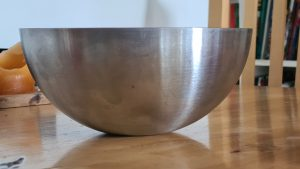I opened the picture in Photoshop, changed the image resolution to match the real dimensions, so to have 20 cm in Photoshop correspond to the width of the bowl in the picture, then proceeded to add guides every 1 cm in width and height, and sampling the bowl curve at every cm.

By doing some curve fitting, I imagined I would be able to find a formula for a hypotetical f(x) function that could approximate a half bowl shape. This required a bit of fiddling, but in the end I got something semi-accurate.Tried several different functions to fit the curve, but the exponential was the smoothest, without artifacts that polynomial functions tend to have. Even though the fit is still not perfect, I thought I’d be good enough. In the end the function that approximates one half of my Ikea bowl is the following:

f(x) = 0.0902209 e 0.458166 xThis function defines the height of the bowl profile given the horizontal coordinate.

Now we can find the area below the curve by calculating the defined integral of this function from 0 to the x coordinate. All we need to do now is to find the points on the x axis that correspond to the equal height layers on the bowl. Let’s call them x1,x2, and x3. The last layer will fill up the bowl completely, so x4 is 10 cm since the bowl’s radius is 10 cm.

Below is an example for the first layer of my bowl, where x1 = 6.76314 cm.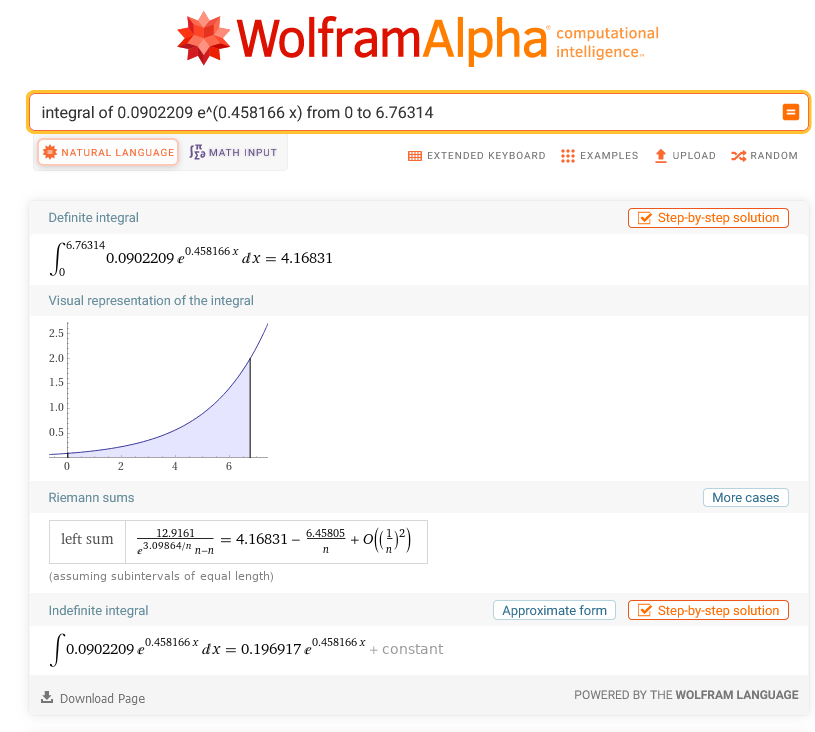I did refresh my derivative and integral rules for this, but ultimately to avoid stupid mistakes and spend another afternoon on it, I again resorted to Wolfram to do this, and the resulting area was then 4.16831 square centimeters.

This is the B area I have marked in my earlier diagram: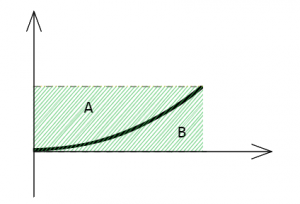Let’s now find A + B, the product of the layer height, which I have set to 2 cm and the x1 coordinate, so:

A + B = 2 cm × x1
= 2 cm × 6.76314 cm
=  13.52628 cm2

We know A + B, and we know B, so we know A as well now.

A = (A + B) – B = 13.52628 cm2 – 4.16831 cm2
A = 9.35797 cm2

Ok, so finally we have the area A of our layer. An area is not a volume though, and we want to know the volume of the liquid or jelly we need to use to fill our first layer.

To do that, we need to calculate the volume of the solid obtained by the rotation (or revolution) of our curve around the y axis. Now, this is easy to visualize if thinking about a cylinder for example, but to calculate the volume of a revolution solid for an arbitrary curve?

I tried several web searches but I could not make much sense of the explanations, sometimes they have errors in the formulas… Once again I get the feeling that I’m too stupid or slow to understand. Sadly, such a feeling has been a constant companion in my life, as a child but not only… The more I grow personally and professionally, the more I think this is often related to the quality of the teaching, articles, text, or paper. In my opinion, some of these materials are not made to be understood, they’re made to make the authors seem smart and competent.

Anyway… while I understand the idea of a revolution solid, the calculations escaped me in this case, so I resorted once again to WolframAlpha, which understands the query directly, if formulated in a way it can digest. In this case the query is:

volume of solid of revolution about the y axis for y = f(x) for x = 0 to x1

If this is not an example of AI, or in Alan Kay’s words,  an amplifier of human intellect, I don’t know what is.

Wolfram calculated a volume for our revolution solid of 128.333 cm3, or 1.28333 dl.
Consider that the volume in question is the volume external to the bowl, underneath it. To get the volume inside the bowl, we need, once again, like in the earlier case of the A and B areas, to subtract this quantity from the volume of an ideal cylinder of height 2 cm and radius = x1 = 6.76314 cm (2 cm is the layer height I chose). If we do that, we obtain:

Vcyl = π × r2 × h = π × 6.763142 × 2 ≈ 287.39329

The internal volume of liquid to fill our bowl to 2 cm of height is then:

Vlayer1 = Vcyl – 128.333 cm3
Vlayer1 = 287.39329 – 128.333 = 159.06029
cm3

This result can’t be correct, can it? It seems too small a volume, there must be something wrong…

Instead of trying to fill the bowl with 159 ml of water, I slightly changed course and tried to calculate the volume of the whole bowl, to see how much water it would contain if it were to be filled to the brim. Following the same method:

Vbowl = Vcyl (h=9, r=10) – Vrev(x=0..10)
with Vcyl (h=9, r=10) = 2827.43339 cm3

which results in the full bowl to have a volume of:

Vbowl = 2827.43339 – 947.447
≈ 1,879.98 cm3

At this point, I felt the anticipation of a child waiting for a birthday cake. I was a bit doubtful a 20 cm bowl could hold almost 1.9 liters of water… nevertheless I took a container big enough, filled it with about 1879 grams of water, and then slowly poured the water in the ikea bowl.

I watched as the water almost immediately filled the bowl up to a half. I still had so much left that it would seem impossible but, to my excitement and complete amazement, the bowl ate up all the water, and there was not even a millimeter of height left.

Oh the absolute joy I felt in that moment! I started screaming from the excitement, my family thought I lost my mind for a minute :-) Such a nerdy thing, but so cool!

If you ask me, this is what the joy of mathematics (if you consider this mathematics, maybe it’s more engineering?) should be all about! In my opinion, this is a perfect example of what we should be teaching our children when we teach them mathematics. It was an ultimately pointless, but so intellectually satisfying achievement. I loved it!

Of course, I wasn’t done with this yet. I used the exact same procedure to calculate the volume of the other 2 cm high layers, and it turned out the first layer indeed consists of a very small volume, and the higher in the bowl you go, the more water is “absorbed” by the upper layers.

Why write this long and very boring post?

I wanted to share this positive feeling with others that, like me, may think of themselves that they don’t “get” math, they were never good in school, or maybe – like me – they were taught mathematics in a joyless, boring, mechanical or too abstract way, being completely deprived of the satisfaction that comes from the discovery and application of math to the real world.

I now wonder, perhaps there is already a website for cakes or similar that lets you calculate the volume of these layers, for different bowls, maybe with proportions of recipes, … who knows?
If you enjoyed this, I’d love to hear from you.

Thanks to Excalidraw and WolframAlpha for being awesome tools one can use to calculate layers of a bowl. Like and subscribe for more :-)